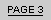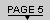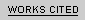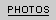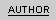M O D E R N I T Y   A N D   T H E   M A N I A CP a g e   F i v e :   J a n e t   L e i g h   a s   I c o n A f t e r   s h e   i s   a t t a c k e d,   M a r i o n   s l u m p s   d e a d   t o   t h e   b a t h r o o m   f l o o r .A R T I C L E   B Y   R I C H A R D   A R M S T R O N G